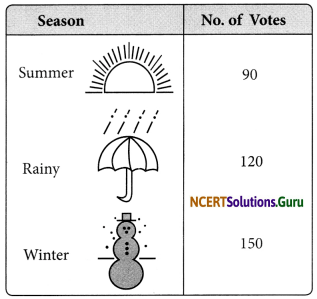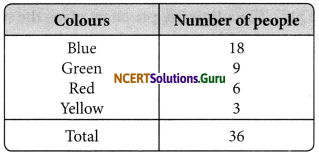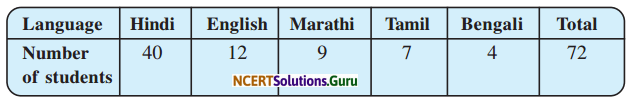# NCERT Solutions for Class 8 Maths Chapter 5 Data Handling Ex 5.2

These NCERT Solutions for Class 8 Maths Chapter 5 Data Handling Ex 5.2 Questions and Answers are prepared by our highly skilled subject experts.

## NCERT Solutions for Class 8 Maths Chapter 5 Data Handling Exercise 5.2

Question 1.
A survey was made to find the type of music that a certain group of young people liked in a city. The adjoining pie chart shows the findings of this survey. From this pie chart answer the following:
(i) If 20 people liked classical music, how many young people were surveyed?
(ii) Which type of music is liked by the maximum number of people?
(iii) If a cassette company were to make 1000 CD’s how many of each type would they make?Solution:
Let the required number of young people be ‘x’
∴ 10% of x = 20
$$\frac{10}{100}$$ × x = 20
x = $$\frac{20 \times 100}{10}$$ = 200
(ii) Light music is liked by the maximum number of people
(iii) Total number of CD’s = 1000
Number of CD’s of Semi classical music = 20% of 1000
= $$\frac{20}{100}$$ × 1000
= 200
Number of CD’s for classical = 10% of 1000
= $$\frac{10}{100}$$ × 1000
= 100
Number of CD’s for folks = 30% of 1000
= $$\frac{30}{100}$$ × 1000
= 300
Number of CD’s for light music = 40% of 1000
= $$\frac{40}{100}$$ × 1000
= 400Question 2.
A group of 360 people were asked to vote for their favourite season from the three season rainy, winter and summer.(i) Which season got the most votes?
(ii) Find the central angle of each sector.
(iii) Draw a pie chart to show this information.
Solution:
(i) Winter season got the most votes.
(ii) Total votes = 90 + 120 + 150 = 360
Central angle of the sector corresponding to summer season
No. of people who vote for summer
= $$\frac{\text { No. of people who vote for summer }}{\text { No. of people }} \times 360$$
= $$\frac{90}{360}$$ × 360°
= 90°
rainy sector = $$\frac{120}{360}$$ × 360° = 120°
winter sector = $$\frac{150}{360}$$ × 360° = 150°Question 3.
Draw a pie chart showing the following information. The table shows the colours preferred by a group of people.Find the proportion of each sector. For example, Blue is $$\frac{18}{36}=\frac{1}{2}$$; Green is $$\frac{9}{36}=\frac{1}{4}$$ and so on. Use this to find the corresponding angles.
Solution:The required pie chart is given below:Question 4.
The adjoining pie chart gives the marks scored in an examination by a student in Hindi, English, Mathematics, Social Science and Science. If the total marks obtained by the students were 540, answer the following questions.(i) In which subject did the student score 105 marks? (Hint: for 540 marks, the central angle = 360°. So, for 105 marks, what is the central angle?)
(ii) How many more marks were obtained by the student in Mathematics than in Hindi?
(iii) Examine whether the sum of the marks obtained in Social Science and Mathematics is more than in Science and Hindi. (Hint: Just study the central angles).
Solution:
(i) Total marks = 540
∴ For 540 marks, the central angle = 360°
∴ For 105 marks, the central angle = $$\frac {360}{540}$$ × 105 = 70°
Since the sector having central angle 70° is corresponding to Hindi. Thus, the student scored 105 marks in Hindi.
(ii) Marks obtained in Mathematics = $$\frac{90^{\circ}}{360^{\circ}} \times 540$$ = 135
Students in Mathematics scored = 135 – 105 = 30
(iii) Sum of the central angles for social science and mathematics = 65° + 90° = 155°
Sum of the central angles for science and Hindi = 80 + 70 = 150°
∴ Marks obtained are proportional to the central angles corresponding to various items and 155° > 150°
∴ Marks obtained in Science and Mathematics are more than the marks obtained in Science and Hindi.Question 5.
The number of students in a hostel, speaking different languages is given below. Display the data in a pie chart.Solution:
Central angle of the sector representing
(a) Hindi language = $$\frac{40}{72}$$ × 360° = 200°
(b) English language = $$\frac{12}{72}$$ × 360° = 60°
(c) Marathi Language = $$\frac{9}{72}$$ × 360° = 45°
(d) Tamil language = $$\frac{7}{72}$$ × 360° = 35°
(e) Bengali language = $$\frac{4}{72}$$ × 360 = 20°
Thus, the required pie chart is given below.error: Content is protected !!